Martin McBride
2022-05-30

The gradient measures the slope of a line.

To find the gradient of a line, we need to know two points on the line. We can then work out the gradient from the formula:

$$gradient = \frac{change\ in\ y}{change\ in\ x}$$

We will find the gradient of this line: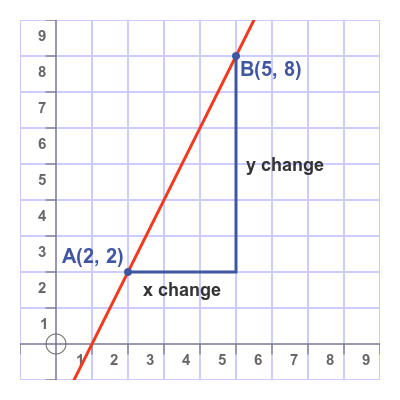We have marked two points on the line:

• A (2, 2)
• B (5, 8)

It is important to use accurate points, otherwise, the gradient will not be accurate. We have chosen two points where the line passes through the grid of the graph paper, so the coordinates are exact whole numbers.

We find the change in x by subtracting the x-coordinate of A (which is 2) from the x-coordinate of B (which is 5):

$${change\ in\ x} = 5 - 2 = 3$$

We find the change in y by subtracting the y-coordinate of A (which is 2) from the y-coordinate of B (which is 8):

$${change\ in\ y} = 8 - 2 = 6$$

So we can calculate the gradient using the formula:

$$gradient = \frac{change\ in\ y}{change\ in\ x} = \frac{6}{3} = 2$$

The gradient is positive, which means that the line goes uphill.

This line goes downhill, so we would expect it to have a negative gradient: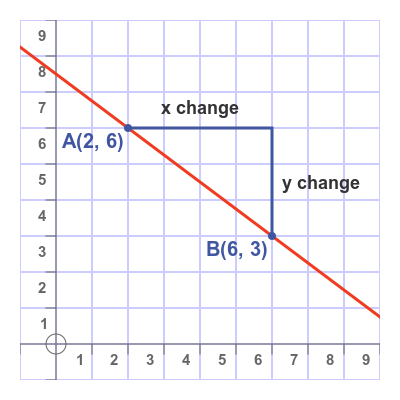Here are two points on the line:

• A (2, 6)
• B (6, 3)

Again we find the change in x by subtracting the x-coordinate of A (which is 2) from the x-coordinate of B (which is 6):

$${change\ in\ x} = 6 - 2 = 4$$

And we find the change in y by subtracting the y-coordinate of A (which is 6) from the y-coordinate of B (which is 3):

$${change\ in\ y} = 3 - 6 = -3$$

We must subtract A from B. Don't make the mistake of always subtracting the smallest from the largest! These values give a gradient of:

$$gradient = \frac{change\ in\ y}{change\ in\ x} = \frac{-3}{4} = -0.75$$

This time the gradient is negative, which means that the line goes downhill.

## Check that the sign is correct

If you always subtract A from B for both x and y, you should always find that the value is positive for uphill curves, and negative for downhill curves.

But it is always worth checking. For example, if you calculate a positive gradient when the curve has a downhill slope, then you have made a mistake. Check that you have done the subtractions the right way round.

## What does the gradient measure?

The gradient tells us how much y changes if we change x by one.

## Special cases

We previously looked at horizontal and vertical lines. What gradients do those lines have?

Here is a horizontal line: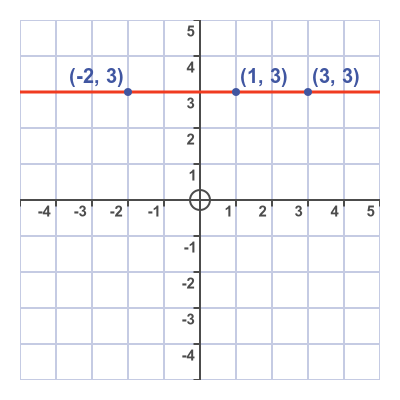If we apply the formula to two of the points, (1, 3) and (3, 3), the result is:

$$gradient = \frac{change\ in\ y}{change\ in\ x} = \frac{3-3}{3-1} = 0$$

The gradient is zero. The line is neither uphill nor downhill, and zero is neither positive nor negative.

Here is a vertical line: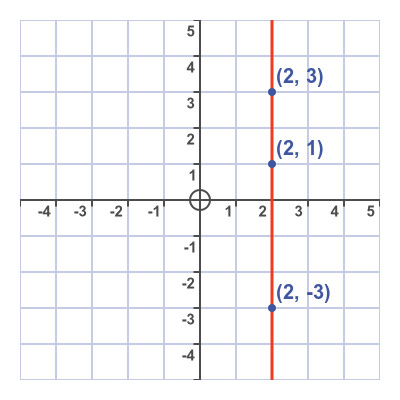If we apply the formula to two of the points, (2, 1) and (2, 3), the result is:

$$gradient = \frac{change\ in\ y}{change\ in\ x} = \frac{3-1}{2-2} = \frac{2}{0}$$

We can't divide 2 by 0, so the gradient of a vertical line is undefined.

## Run and rise

The change in x is sometimes called the run, and the change in y is sometimes called the rise: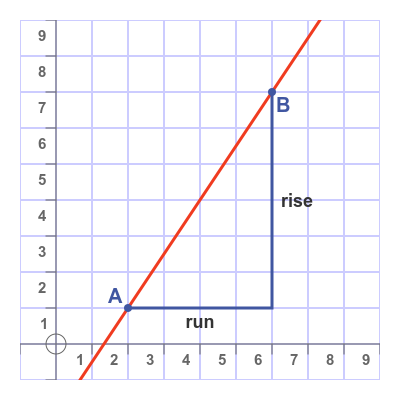These are just different names for the change in x and y. The gradient is then given by:

$$gradient = \frac{rise}{run}$$

For negative gradients, the change in y is usually called the fall.